# Class 11 NCERT Solutions – Chapter 6 Linear Inequalities – Exercise 6.2

### Question 1: x + y < 5

Solution:

Now draw a dotted line x + y = 5 in the graph (because (x + y = 5) is NOT the part of the given equation)

We need at least two solutions of the equation. So, we can use the following table to draw the graph:

Consider x + y < 5

Lets, select origin point (0, 0)

⇒ 0 + 0 < 5

⇒ 0 < 5 (this is true)

Hence, Solution region of the given inequality is the line x + y = 5. where, Origin is included in the region

The graph is as follows: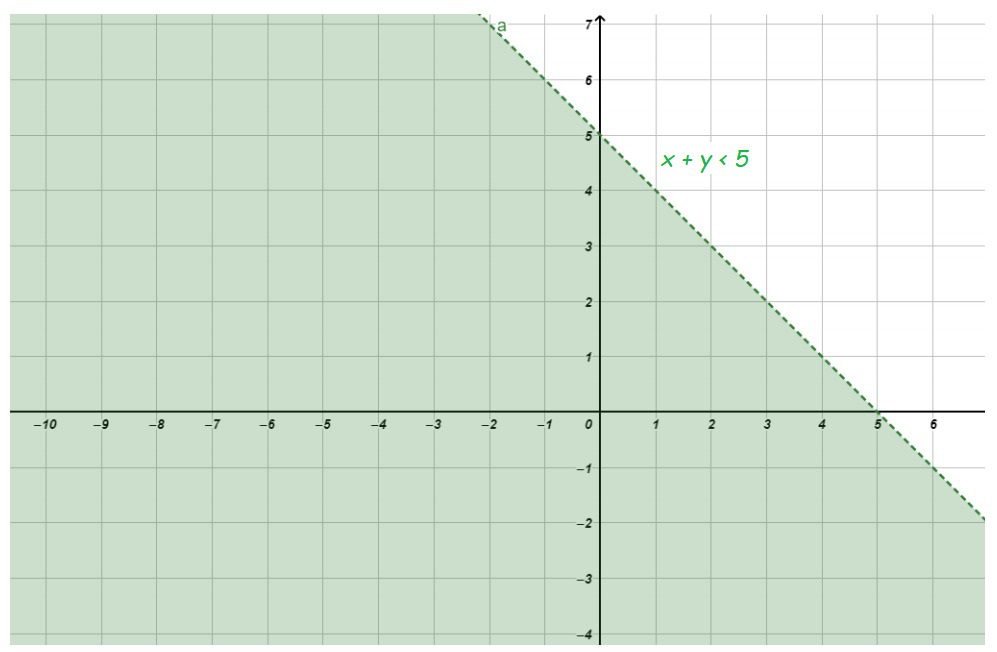### Question 2: 2x + y ≥ 6

Solution:

Now draw a solid line 2x + y = 6 in the graph (because (2x + y = 6) is the part of the given equation)

We need at least two solutions of the equation. So, we can use the following table to draw the graph:

Consider 2x + y ≥ 6

Lets, select origin point (0, 0)

⇒ 0 + 0 ≥ 6

⇒ 0 ≥ 6 (this is not true)

Hence, Solution region of the given inequality is the line 2x + y ≥ 6. where, Origin is not included in the region

The graph is as follows: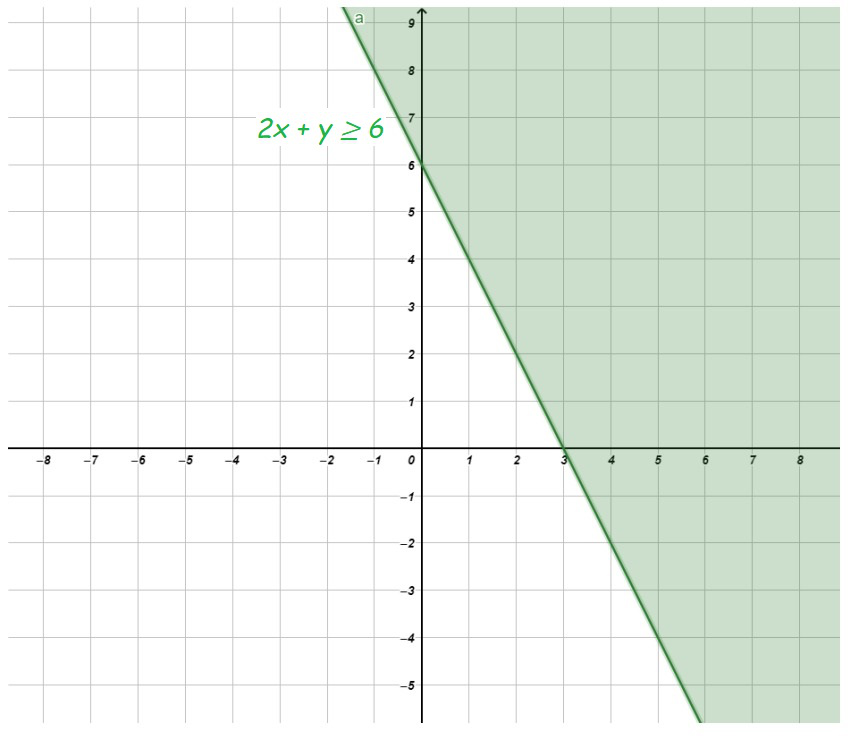### Question 3: 3x + 4y ≤ 12

Solution:

Now draw a solid line 3x + 4y = 12 in the graph (because (3x + 4y = 12) is the part of the given equation)

We need at least two solutions of the equation. So, we can use the following table to draw the graph:

Consider 3x + 4y ≤ 12

Lets, select origin point (0, 0)

⇒ 0 + 0 ≤ 12

⇒ 0 ≤ 12 (this is true)

Hence, Solution region of the given inequality is the line 3x + 4y ≤ 12. where, Origin is included in the region

The graph is as follows: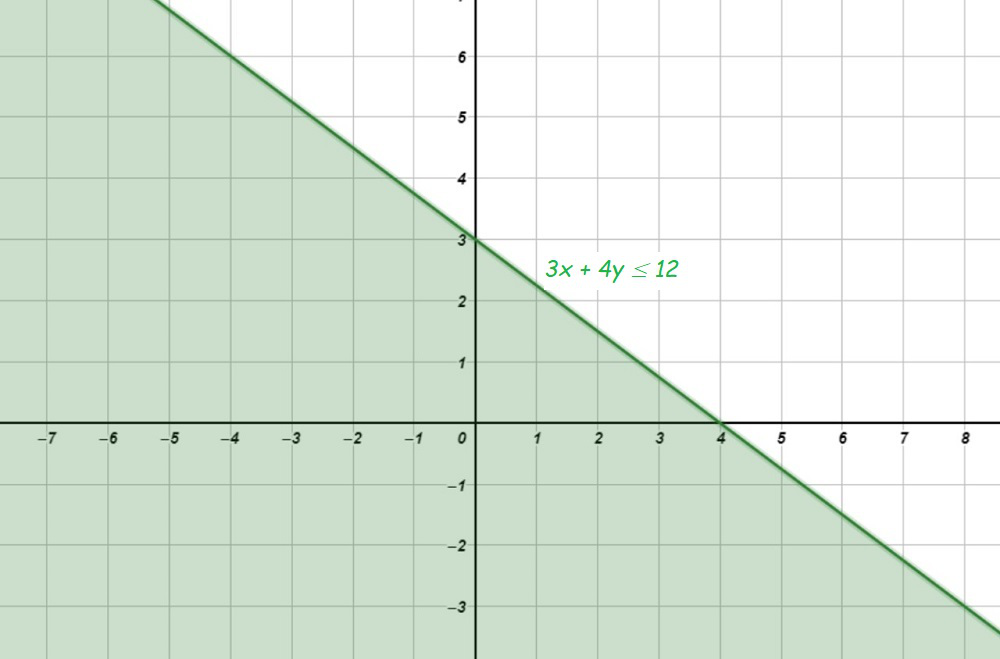### Question 4: y + 8 ≥ 2x

Solution:

Now draw a solid line y + 8 = 2x in the graph (because (y + 8 = 2x) is the part of the given equation)

We need at least two solutions of the equation. So, we can use the following table to draw the graph:

Consider y + 8 ≥ 2x

Lets, select origin point (0, 0)

⇒ 0 + 8 ≥ 0

⇒ 8 ≥ 0 (this is true)

Hence, Solution region of the given inequality is the line y + 8 ≥ 2x. where, Origin is included in the region

The graph is as follows: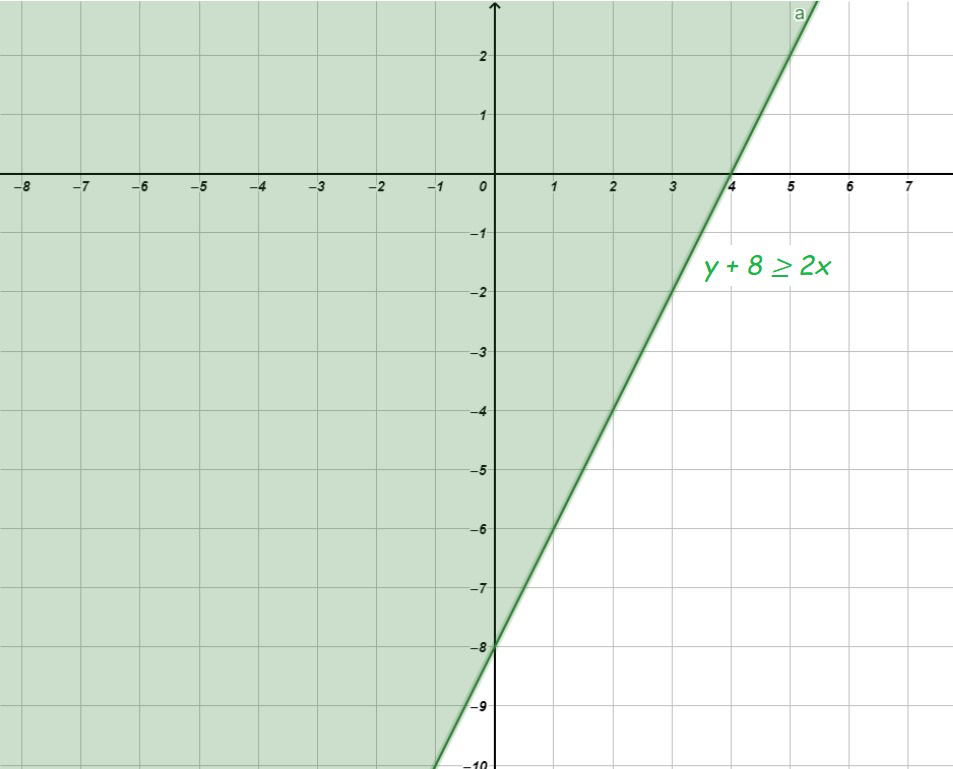### Question 5: x – y ≤ 2

Solution:

Now draw a solid line x – y = 2 in the graph (because (x – y = 2) is the part of the given equation)

We need at least two solutions of the equation. So, we can use the following table to draw the graph:

Consider x – y ≤ 2

Lets, select origin point (0, 0)

⇒ 0 – 0 ≤ 2

⇒ 0 ≤ 2  (this is true)

Hence, Solution region of the given inequality is the line x – y ≤ 2. where Origin is included in the region

The graph is as follows: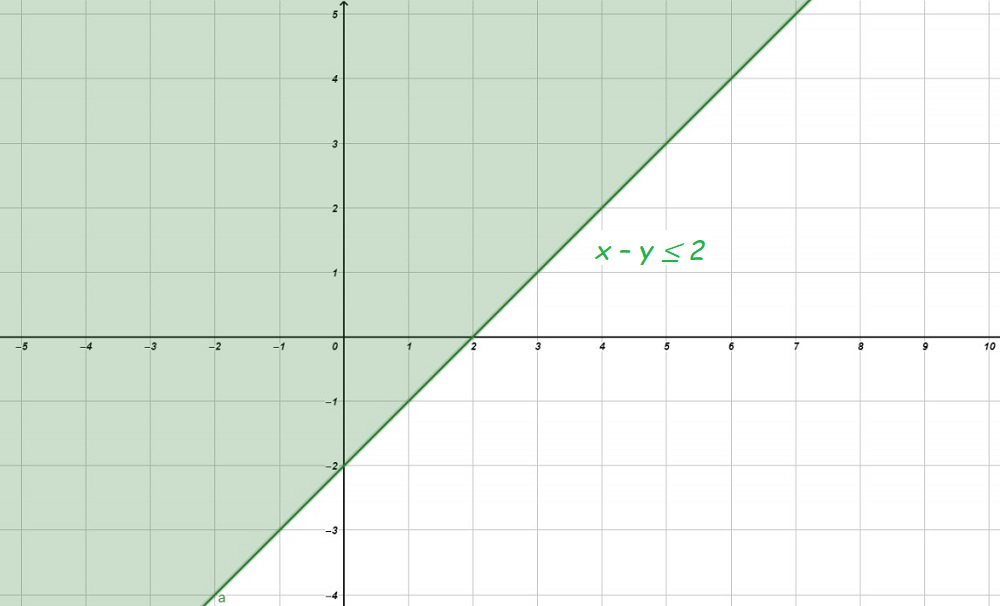### Question 6: 2x – 3y > 6

Solution:

Now draw a dotted line 2x – 3y = 6 in the graph (because (2x – 3y = 6) is NOT the part of the given equation)

We need at least two solutions of the equation. So, we can use the following table to draw the graph:

Consider 2x – 3y > 6

Lets, select origin point (0, 0)

⇒ 0 + 0 > 6

⇒ 0 > 6 (this is not true)

Hence, Solution region of the given inequality is the line 2x – 3y > 6. where, Origin is not included in the region

The graph is as follows: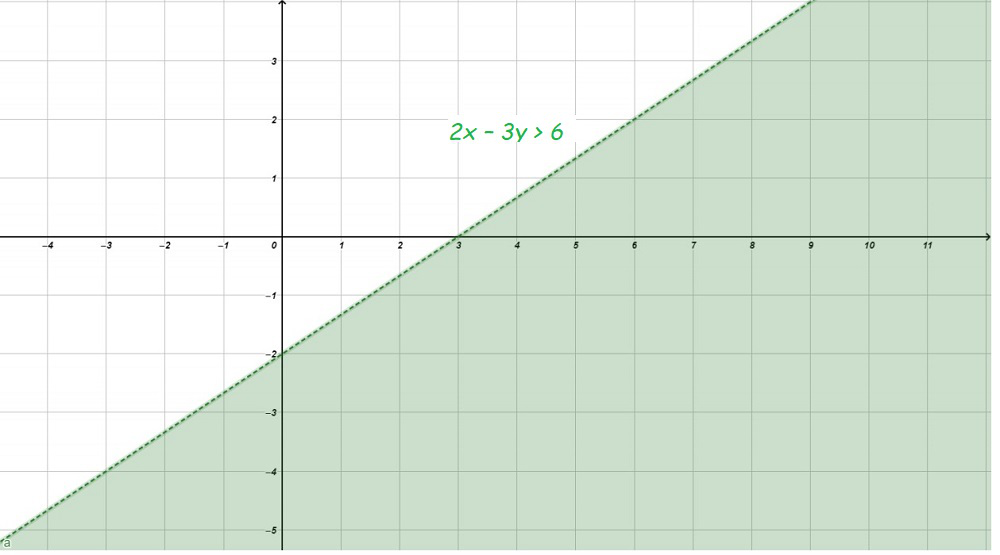### Question 7: – 3x + 2y ≥ – 6

Solution:

Now draw a solid line – 3x + 2y = – 6 in the graph (because (– 3x + 2y = – 6) is the part of the given equation)

We need at least two solutions of the equation. So, we can use the following table to draw the graph:

Consider – 3x + 2y ≥ – 6

Lets, select origin point (0, 0)

⇒ 0 + 0 ≥ – 6

⇒ 0 ≥ – 6 (this is true)

Hence, Solution region of the given inequality is the line – 3x + 2y ≥ – 6. where, Origin is included in the region

The graph is as follows: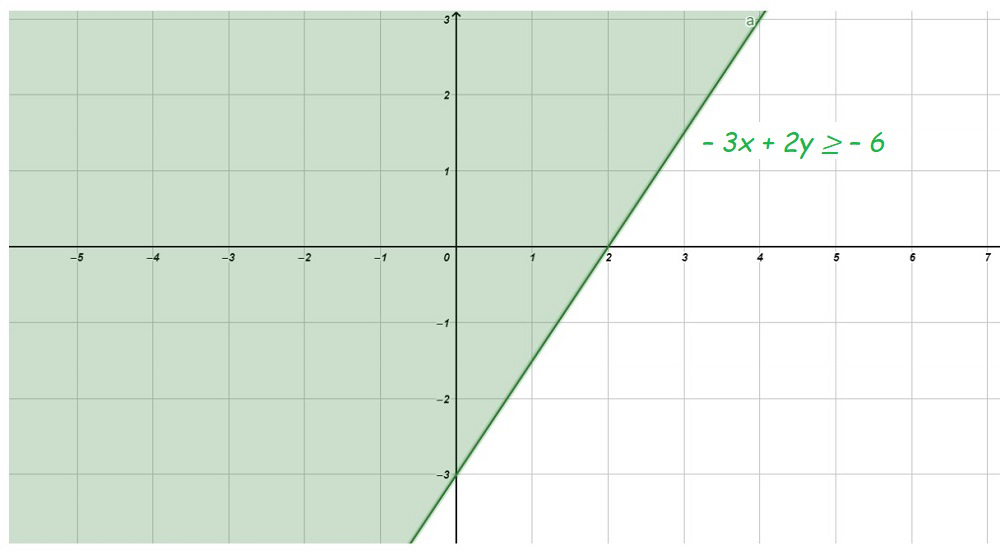### Question 8: 3y – 5x < 30

Solution:

Now draw a dotted line 3y – 5x = 30 in the graph (because (3y – 5x = 30) is NOT the part of the given equation)

We need at least two solutions of the equation. So, we can use the following table to draw the graph:

Consider 3y – 5x < 30

Lets, select origin point (0, 0)

⇒ 0 + 0 < 30

⇒ 0 < 30 (this is true)

Hence, Solution region of the given inequality is the line 3y – 5x < 30. where, Origin is included in the region

The graph is as follows: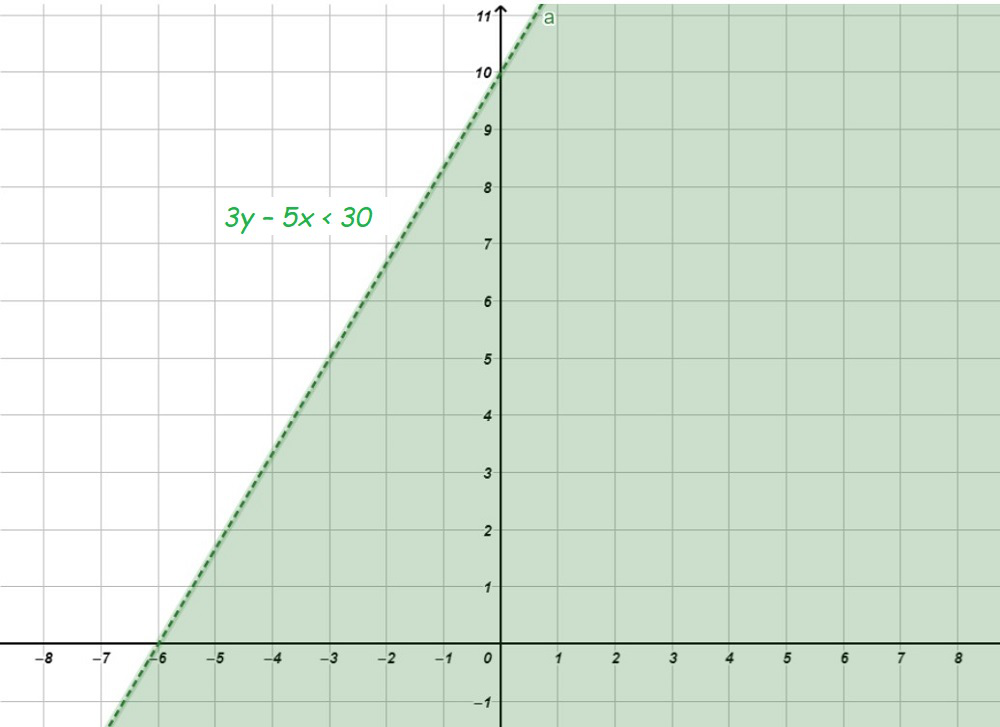### Question 9: y < – 2

Solution:

Now draw a dotted line y = – 2 in the graph (because (y = – 2) is NOT the part of the given equation)

we need at least two solutions of the equation. So, we can use the following table to draw the graph:

Consider y < – 2

Lets, select origin point (0, 0)

⇒ 0 < – 2

⇒ 0 < – 2 (this is not true)

Hence, Solution region of the given inequality is the line y < – 2. where, Origin is not included in the region

The graph is as follows: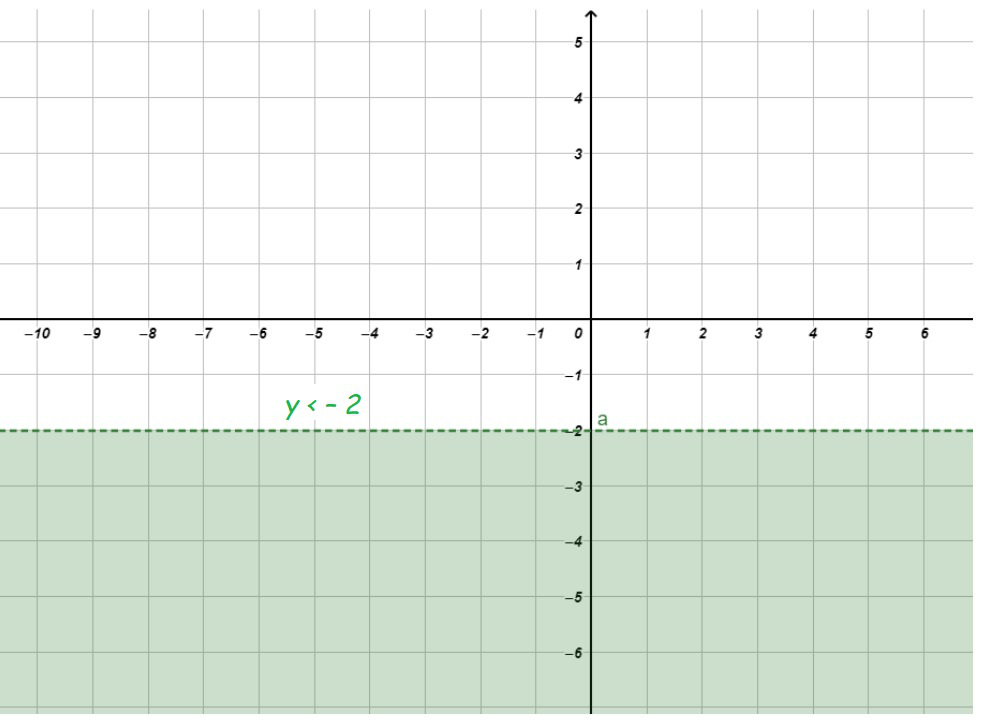### Question 10: x > – 3

Solution:

Now draw a dotted line x = – 3 in the graph (because (x = – 3) is NOT the part of the given equation)

we need at least two solutions of the equation. So, we can use the following table to draw the graph:

Consider x > – 3

Lets, select origin point (0, 0)

⇒ 0 > – 3

⇒ 0 > – 3 (this is true)

Hence, Solution region of the given inequality is the line x > – 3. where, Origin is included in the region

The graph is as follows: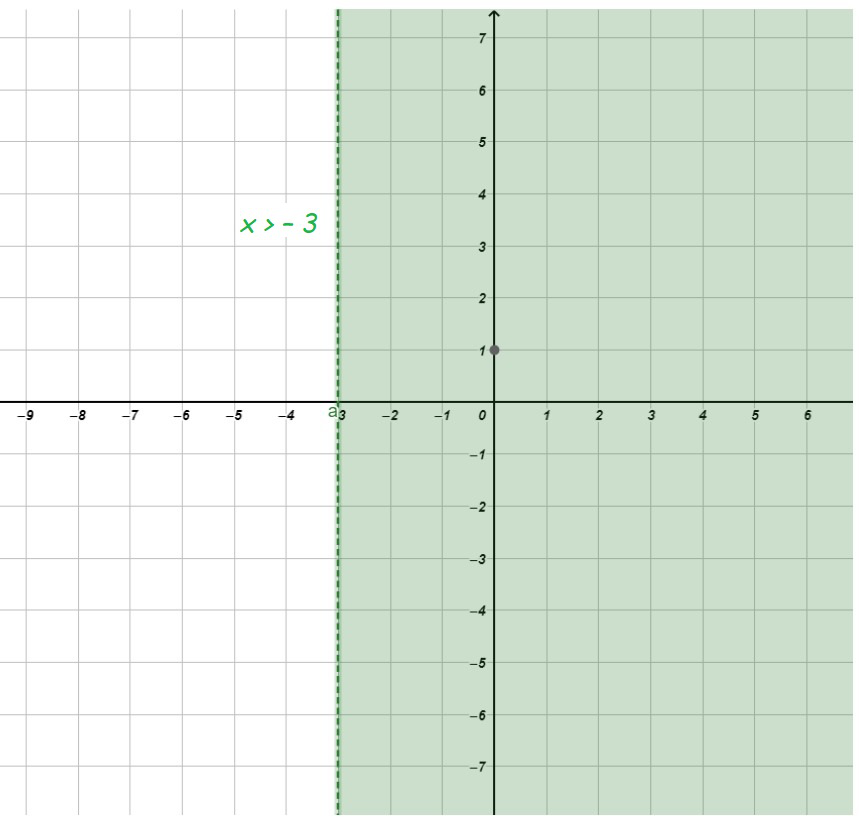Whether you're preparing for your first job interview or aiming to upskill in this ever-evolving tech landscape, GeeksforGeeks Courses are your key to success. We provide top-quality content at affordable prices, all geared towards accelerating your growth in a time-bound manner. Join the millions we've already empowered, and we're here to do the same for you. Don't miss out - check it out now!

Previous
Next